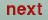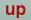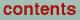Next: Organization of TB-LMTO Programs Up: The STUTTGART TB-LMTO-ASA program. Previous: Installation of the TB-LMTO

# Execution of the TB-LMTO programs

1. The basic input data is inserted in the CTRL file. The CTRL file shown below contains the minimum amount of information to be typed, in order to run the TB-LMTO programs. The rest may be obtained from the default values in the program, which are written into the CTRL file by the program.
```HEADER  NiSe2 Pyrit (Acta Chem. Scand.
v. 23 p. 2325 1969)
VERS    LMASA-47
STRUC   ALAT=11.2683
PLAT=1 0 0
0 1 0
0 0 1
CLASS   ATOM=NI Z=28
ATOM=Se Z=34
SITE    ATOM=NI POS= .0  .0  .0
ATOM=NI POS= .5  .0  .5
ATOM=NI POS= .0  .5  .5
ATOM=NI POS= .5  .5  .0
ATOM=Se POS= .383  .383  .383
ATOM=Se POS= .617  .617  .617
ATOM=Se POS= .117  .617  .883
ATOM=Se POS= .883  .383  .117
ATOM=Se POS= .617  .883  .117
ATOM=Se POS= .383  .117  .883
ATOM=Se POS= .883  .117  .617
ATOM=Se POS= .117  .883  .383```

The input consists of tokens, like ATOM= or Z=. These are grouped together in categories, like CLASS. The name of a category always starts in the first column of a line. This will be explained in detail in Section V. ALAT= is a lattice constant in a.u. (i.e. Bohr radii), PLAT= are the lattice translation vectors and POS= are the atomic positions. The latter two are in Cartesian coordinates and in units of ALAT.

All Ni atoms and all Se atoms are equivalent i.e. there are two classes. From the information in the CTRL file, the program will find the symmetry i.e. all space group operations and the generators of the space group. If the symmetry of the bravais lattice is incompatible with the symmetry of the basis, then the symmetry of the bravais lattice is used. Alternatively to the CTRL file above, the symmetry generators and only one of each of the inequivalent atoms could have been given. The program will complete the basis:

```HEADER  NiSe2 Pyrit (Acta Chem. Scand.
v. 23 p. 2325 1969)
VERS    LMASA-47
STRUC   ALAT=11.2683
PLAT=1 0 0
0 1 0
0 0 1
CLASS   ATOM=NI Z=28
ATOM=Se Z=34
SITE    ATOM=NI POS= .0  .0  .0
ATOM=Se POS= .383  .383  .383
SYMGRP  NGEN=3 GENGRP=I R3D MX:(.5,.5,0)```

The category SYMGRP contains three tokens: NGEN= the number of generators supplied,
GENGRP= the names of the generators as defined in the Subsection `Category SYMGRP'.

A third possibility is to use the interactive program lminit.run in the main directory. An example is shown below, where the user's answer to the query is indicated by the string after `->' and followed by `(Answer)'.

```| QUERY : SPCGRP:
|         SYMBOL OR NUMBER OF SPACE GROUP
|
| Enter: 'nnn', nnn is the value for SPCGRP,
|         'a' to abort program execution.

Space group: Pa-3       No.:205
Crystal system: cubic
Generators: I R3D MX::(1/2,1/2,0)

| QUERY :  atomic-units ? (else in Angstrom)
|
| Enter: 'nnn', nnn is the value for ATUNITS,
|               default value=T
|         'a' to abort program execution.
|         '/' to accept default value
|        ->/

Now enter lattice parameters

| QUERY : A:lattice constant (atomic units)
|
| Enter: 'nnn', nnn is the value for A,
|         'a' to abort program execution.

CTRLUC:             PLAT   ALAT=11.2683
1.00000    .00000    .00000
.00000   1.00000    .00000
.00000    .00000   1.00000

| QUERY: label or nuclear charge of class 1
|
| Enter: 'nnn', nnn is the value for label,
|         'a' to abort program execution.
|         'q' to quit

Class: Ni  ; nuclear charge 28 (atom Ni)

| QUERY: X = position of Ni (in units of the
|         translation vectors a, b, c)
|
| Enter: 'nnn', nnn is the value for X,
|               default value=0. 0. 0.
|         'a' to abort program execution.
|         '/' to accept default value

| QUERY: label or nuclear charge of class 2
|
| Enter: 'nnn', nnn is the value for label,
|         'a' to abort program execution.
|         'q' to quit

Class: Se  ; nuclear charge 34 (atom Se)

| QUERY : X = position of Se
|
| Enter: 'nnn', nnn is the value for X,
|               default value=0. 0. 0.
|         'a' to abort program execution.
|         '/' to accept default value
|        ->.383 .383 .383

| QUERY: label or nuclear charge of class 3
|
| Enter: 'nnn', nnn is the value for label,
|         'a' to abort program execution.
|         'q' to quit

CHKSYM: Check the symmetry of the crystal:

GENGRP: A subgroup of the space group will
be created from 3 generators
The generators created 24 sym. ops.

ADDBAS: The basis has been extended from
2 to  12 atoms.
The new positions are:

ATOM=Ni   POS=    .500   .500   .000
ATOM=Ni   POS=    .000   .500   .500
ATOM=Ni   POS=    .500   .000   .500
ATOM=Se   POS=   -.383  -.383  -.383
ATOM=Se   POS=    .117   .883   .383
ATOM=Se   POS=    .883   .117  -.383
ATOM=Se   POS=    .383   .117   .883
ATOM=Se   POS=    .883   .383   .117
ATOM=Se   POS=   -.383   .883   .117
ATOM=Se   POS=    .117  -.383   .883

SYMLAT: lattice invariant under 48 sym. ops.

SYMCRY: crystal invariant under 24 sym. ops.

SHOSYM: Bravais system : cubic
Bravais lattice: cubic primitive
Centring type  : P
Crystal family : cubic
Crystal system : cubic
Point group    : m-3```

The resulting CTRL file looks like this:

```HEADER    Ni4Se8, cubic primitive
SYMGRP    NGEN=3 GENGRP=I R3D MX:(.5,.5,0)
SPCGRP=Pa-3
STRUC     ALAT=11.2683
PLAT=1 0 0
0 1 0
0 0 1
CLASS     ATOM=Ni Z=28
ATOM=Se Z=34
SITE      ATOM=Ni POS=0.000 0.000 0.000
ATOM=Ni POS=0.500 0.500 0.000
ATOM=Ni POS=0.500 0.000 0.500
ATOM=Ni POS=0.000 0.500 0.500
ATOM=Se POS=0.383 0.117 -.117
ATOM=Se POS=-.383 -.117 0.117
ATOM=Se POS=-.117 0.383 0.117
ATOM=Se POS=0.117 -.383 -.117
ATOM=Se POS=0.117 -.117 0.383
ATOM=Se POS=-.117 0.117 -.383
ATOM=Se POS=0.383 0.383 0.383
ATOM=Se POS=-.383 -.383 -.383```
2. The next step is to find the size of the atomic spheres. First muffin-tin (MT) radii are found by lmhart.run and inserted into the CTRL file. The result of this run is shown below.
```HEADER    Ni4Se8, cubic primitive
VERS      LMASA-47
IO        VERBOS=30 OUTPUT=LM ERR=ERR
SYMGRP    NGEN=3 GENGRP=I R3D MX:(.5,.5,0)
SPCGRP=Pa-3
STRUC     ALAT=11.2683
PLAT=1 0 0
0 1 0
0 0 1
DIM       NBAS=12 NCLASS=2 NL=3 LDIM=68
IDIM=40 NSYMOP=24
CLASS     ATOM=Ni Z=28 R=2.41776510
ATOM=Se Z=34 R=2.28352037
SITE      ATOM=Ni POS=0.000 0.000 0.000
ATOM=Ni POS=0.500 0.500 0.000
ATOM=Ni POS=0.500 0.000 0.500
ATOM=Ni POS=0.000 0.500 0.500
ATOM=Se POS=0.383 0.117 -.117
ATOM=Se POS=-.383 -.117 0.117
ATOM=Se POS=-.117 0.383 0.117
ATOM=Se POS=0.117 -.383 -.117
ATOM=Se POS=0.117 -.117 0.383
ATOM=Se POS=-.117 0.117 -.383
ATOM=Se POS=0.383 0.383 0.383
ATOM=Se POS=-.383 -.383 -.383```

The two tokens (R=) with the MT-radii in a.u. have been inserted in category CLASS. Furthermore, a new class, IO, has been inserted with the tokens: VERBOS= which specifies the amount of output the programs print and the number of default tokens which will be inserted in the CTRL file by the programs which updates the CTRL file, OUTPUT= which gives a file name to which standard out will be directed, ERR= which gives a file name to which error messages will be directed.

3. The overlap between the WS-spheres is checked by the lmovl.run program. The result written to standard out looks like (the output has been edited to fit into this documentation):
```Info: impossible to reach VOL, increase OMMAX.

CELL VOLUME= 1430.78771,
SUM OF SPHERE VOLUMES=   992.45473

IB JB  CL1  CL2  DIST SUMRS  1/d 1/s1 1/s2
==========================================
1  5  Ni   Se    .42 .48    16  27   28
------------------------------------------
5  1  Se   Ni    .42 .48    16  28   27
5  6  Se   Se    .41 .47    16  28   28
------------------------------------------```

The first line tells that space filling could not be reached with the default maximum overlaps (two atomic spheres are allowed to overlap no more than 16% in the sence of 1/d below, max. overlap between an atomic and an interstitial sphere is 18%, and max. overlap between two interstitial spheres is 20%).

The next two lines show the cell volume and the volume inside the expanded spheres.

In the table, each line displays two neighboring atoms with WS-radii s1 and s2, and the distance between them d. Under `1/d', `1/s1', and `1/s2' are the results of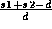,, and, respectively, in percent. If the two sphere radii are very much different, one should check `1/s1', where s1 is the smallest radius. (A big sphere may swallow a small sphere.)

Since in the present case space filling could not be reached, interstitial spheres have to be inserted.

4. Interstitial spheres are found by the lmes.run program. The resulting CTRL file is shown below.
```HEADER Ni4Se8, cubic primitive
VERS   LMASA-47
IO     VERBOS=30 OUTPUT=OVL ERR=ERR
SYMGRP NGEN=3 GENGRP=I R3D MX:(.5,.5,0)
SPCGRP=Pa-3
STRUC  ALAT=11.2683
PLAT=1 0 0
0 1 0
0 0 1
DIM    NBAS=36 NCLASS=3 NL=3 LDIM=68
IDIM=40 NSYMOP=24
CLASS  ATOM=Ni Z=28 R=2.41776510
ATOM=Se Z=34 R=2.28352037
ATOM=E  Z= 0 R=1.53150056
SITE   ATOM=Ni POS=0.00000 0.00000 0.00000
ATOM=Ni POS=0.50000 0.50000 0.00000
ATOM=Ni POS=0.50000 0.00000 0.50000
ATOM=Ni POS=0.00000 0.50000 0.50000
ATOM=Se POS=0.38300 0.11700 -.11700
ATOM=Se POS=-.38300 -.11700 0.11700
ATOM=Se POS=-.11700 0.38300 0.11700
ATOM=Se POS=0.11700 -.38300 -.11700
ATOM=Se POS=0.11700 -.11700 0.38300
ATOM=Se POS=-.11700 0.11700 -.38300
ATOM=Se POS=0.38300 0.38300 0.38300
ATOM=Se POS=-.38300 -.38300 -.38300
ATOM=E  POS=0.28510 -.18060 0.09608
ATOM=E  POS=-.28510 0.18060 -.09608
ATOM=E  POS=0.09608 0.28510 -.18060
ATOM=E  POS=-.09608 -.28510 0.18060
ATOM=E  POS=0.18060 -.09608 -.28510
ATOM=E  POS=-.18060 0.09608 0.28510
ATOM=E  POS=0.31939 0.09608 0.21489
ATOM=E  POS=0.21489 0.31939 0.09608
ATOM=E  POS=-.31939 -.09608 -.21489
ATOM=E  POS=-.21489 -.31939 -.09608
ATOM=E  POS=0.09608 0.21489 0.31939
ATOM=E  POS=-.09608 -.21489 -.31939
ATOM=E  POS=0.40391 -.21489 -.18060
ATOM=E  POS=-.40391 0.21489 0.18060
ATOM=E  POS=-.18060 0.40391 -.21489
ATOM=E  POS=0.18060 -.40391 0.21489
ATOM=E  POS=0.21489 0.18060 -.40391
ATOM=E  POS=-.21489 -.18060 0.40391
ATOM=E  POS=0.40391 -.28510 0.31939
ATOM=E  POS=0.31939 0.40391 -.28510
ATOM=E  POS=-.40391 0.28510 -.31939
ATOM=E  POS=-.31939 -.40391 0.28510
ATOM=E  POS=-.28510 0.31939 0.40391
ATOM=E  POS=0.28510 -.31939 -.40391```

The program found 24 interstitial spheres, all equivalent, with a sphere radius between 0.9 and 4.0 a.u. These limits are default and can be changed. Notice that a new category DIM has been inserted, which contain information about some dimensions.

5. Check the overlap again with lmovl.run. Standard out now looks like:
```IB  JB  CL1  CL2 DIST SUMRS 1/d 1/s1 1/s2
=========================================
1   5  NI   Se  .42  .47    13  22   23
1  20  NI   E1  .35  .40    13  18   29
-----------------------------------------
5   1  Se   NI  .42  .47    13  23   22
5   6  Se   Se  .41  .46    13  23   23
5  14  Se   E1  .34  .38    13  19   28
5  15  Se   E1  .38  .38     1   1    2
5  17  Se   E1  .34  .38    13  19   28
5  22  Se   E1  .34  .38    13  19   28
5  23  Se   E1  .34  .38    13  19   28
5  29  Se   E1  .38  .38     1   1    2
5  31  Se   E1  .34  .38    13  19   28
5  34  Se   E1  .34  .38    13  19   28
5  35  Se   E1  .38  .38     1   1    2
5  36  Se   E1  .34  .38    13  19   28
-----------------------------------------
13   2  E1   NI  .35  .40    13  29   18
13   6  E1   Se  .34  .38    13  28   19
13   7  E1   Se  .38  .38     1   2    1
13   8  E1   Se  .34  .38    13  28   19
13  12  E1   Se  .34  .38    13  28   19
13  15  E1   E1  .27  .31    12  21   21
13  25  E1   E1  .30  .31     1   2    2
-----------------------------------------```

The program found space filling with a maximum overlap of 13% which is fine and one can proceed.

6. Complete the CTRL file with the lmctl.run program, but first change the token VERBOS= to 50 in category IO to ensure that all default values are inserted.
```HEADER  Ni4Se8, cubic primitive
VERS    LMASA-47
IO      VERBOS=50 HELP=F WKP=F IACTIV=F
ERRTOL=2 OUTPUT=CTL ERR=ERR
SYMGRP  NGEN=3 GENGRP=I R3D MX:(.5,.5,0)
SPCGRP=Pa-3 USESYM=F
STRUC   ALAT=11.2683
PLAT=1 0 0
0 1 0
0 0 1 FIXLAT=T
DIM     NBAS=36 NCLASS=3 NL=3 LDIM=92
IDIM=232 NSYMOP=24 NKP=11
OPTIONS NSPIN=1 REL=T CCOR=T NONLOC=F
NRXC=1 NRMIX=2 CORDRD=F
NITATOM=30 CHARGE=F FATBAND=F AFM=F
FS=F CARTESIAN=T WRIBAS=F Q=----
CLASS   ATOM=Ni Z=28 R=2.72719158 LMX=2
CONF=4 4 3 4 IDXDN=1 1 1 IDMOD=0 0 0
ATOM=Se Z=34 R=2.57576616 LMX=2
CONF=4 4 4 4 IDXDN=1 1 2 IDMOD=0 0 0
ATOM=E  Z= 0 R=1.72750257 LMX=2
CONF=1 2 3 4 IDXDN=1 2 2 IDMOD=0 0 0
SITE    ATOM=Ni POS=0.00000 0.00000 0.0000
ATOM=Ni POS=0.50000 0.50000 0.0000
ATOM=Ni POS=0.50000 0.00000 0.5000
ATOM=Ni POS=0.00000 0.50000 0.5000
ATOM=Se POS=0.38300 0.11700 -.1170
ATOM=Se POS=-.38300 -.11700 0.1170
ATOM=Se POS=-.11700 0.38300 0.1170
ATOM=Se POS=0.11700 -.38300 -.1170
ATOM=Se POS=0.11700 -.11700 0.3830
ATOM=Se POS=-.11700 0.11700 -.3830
ATOM=Se POS=0.38300 0.38300 0.3830
ATOM=Se POS=-.38300 -.38300 -.3830
ATOM=E  POS=0.28510 -.18060 0.0960
ATOM=E  POS=-.28510 0.18060 -.0960
ATOM=E  POS=0.09608 0.28510 -.1806
ATOM=E  POS=-.09608 -.28510 0.1806
ATOM=E  POS=0.18060 -.09608 -.2851
ATOM=E  POS=-.18060 0.09608 0.2851
ATOM=E  POS=0.31939 0.09608 0.2148
ATOM=E  POS=0.21489 0.31939 0.0960
ATOM=E  POS=-.31939 -.09608 -.2148
ATOM=E  POS=-.21489 -.31939 -.0960
ATOM=E  POS=0.09608 0.21489 0.3193
ATOM=E  POS=-.09608 -.21489 -.3193
ATOM=E  POS=0.40391 -.21489 -.1806
ATOM=E  POS=-.40391 0.21489 0.1806
ATOM=E  POS=-.18060 0.40391 -.2148
ATOM=E  POS=0.18060 -.40391 0.2148
ATOM=E  POS=0.21489 0.18060 -.4039
ATOM=E  POS=-.21489 -.18060 0.4039
ATOM=E  POS=0.40391 -.28510 0.3193
ATOM=E  POS=0.31939 0.40391 -.2851
ATOM=E  POS=-.40391 0.28510 -.3193
ATOM=E  POS=-.31939 -.40391 0.2851
ATOM=E  POS=-.28510 0.31939 0.4039
ATOM=E  POS=0.28510 -.31939 -.4039
SCALE   SCLWSR=T OMMAX1=.16 .18 .20
OMMAX2=.40 .45 .50
STR     KAPPA2=0 RMAXS=3.2 NDIMIN=350
NOCALC=F IALPHA=0
DOWATS=F DELTR=.1 LMAXW=8
ATOM=Ni SIGMA=.7 .7 .7
ATOM=Se SIGMA=.7 .7 .7
ATOM=E  SIGMA=.7 .7 .7
START   NIT=30 BROY=T WC=-1 NMIX=1 BETA=.5
FREE=F CNVG=.00001 CNVGET=.00001
BEGMOM=T CNTROL=T
EFERMI=-.25 VMTZ=-.75
ATOM=Ni  P=4.67 4.41 3.86
Q=0.6 0.0 0.0
0.8 0.0 0.0
8.6 0.0 0.0
enu   =-.543 -.398 -.304
c     =-.419 0.544 -.281
sqrdel=0.464 0.580 0.188
p     =0.054 0.027 2.398
gamma =0.546 0.240 -.009
ATOM=Se  P=4.95 4.84 4.17
Q=2.0 0.0 0.0
3.7 0.0 0.0
0.3 0.0 0.0
enu   =-1.005 -0.388 -0.513
c     =-1.304 -0.360  1.311
sqrdel= 0.344  0.387  0.596
p     = 0.372  0.170  0.024
gamma = 0.442  0.145  0.150
ATOM=E   P=1.5 2.5 3.5
Q=0 0 0
0 0 0
0 0 0
enu   =-1.758 -0.642  1.316
c     = 0.990  3.503  6.985
sqrdel= 0.590  0.485  0.400
p     = 0.006  0.003  0.001
gamma = 0.358  0.065  0.022
SPINDENS=F
CHARWIN=F EMIN=-2 EMAX=2
PLOT    ORIGIN=0 0 0
R1=1 0 0 NDELR1=0
R2=0 1 0 NDELR2=0
R3=0 0 1 NDELR3=0
FORMAT=1
BZ      NKABC=4 4 4 TETRA=T METAL=T
TOL=.000001
N=0 W=.005 RANGE=5 NPTS=1001
EWALD   NKDMX=250 AS=2 TOL=.000001
RHOFIT  FIT=F KAPPA2=0 RMAXS=3.5
ATOM=Ni LMXRHO=2 SIGMA=.7 .7 .7
ATOM=Se LMXRHO=2 SIGMA=.7 .7 .7
ATOM=E  LMXRHO=2 SIGMA=.7 .7 .7
SCELL   PLAT=1 0 0
0 1 0
0 0 1 EQUIV=T
HARTREE BEGATOM=T LT1=2 LT2=2 LT3=2
DOS     NOPTS=801 EMIN=-2 EMAX=2
SYML    NQ=30 Q1=0.0 0.0 0.0 LAB1=g
Q2=0.5 0.5 0.0 LAB2=M
NQ=20 Q1=0.5 0.5 0.0 LAB1=M
Q2=0.5 0.0 0.0 LAB2=X
NQ=20 Q1=0.5 0.0 0.0 LAB1=X
Q2=0.0 0.0 0.0 LAB2=g
NQ=35 Q1=0.0 0.0 0.0 LAB1=g
Q2=0.5 0.5 0.5 LAB2=R
FINDES  RMINES=.9 RMAXES=4 NRXYZ=72 72 72```

Notice that the Wigner-Sitz radii have been inserted because SCLWSR=T.

This is a complete CTRL file. The meaning of only a few of these tokens is necessary in order to run the program, however, a complete list with a description of each token is given in SectionV.

7. Before calculating the TB real space structure constants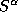and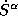for the combined correction term by the lmstr.run program, the verbosity is lowered by changing VERBOS= to 40 in category IO.only depends on the structure and the number of partial waves included, while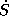also depends on the WS-radii. If these are changed during the calculations, the structure constants have to be recalculated.
8. A self-consistent-field (SCF) calculation can now be performed using the program lm.run. If self-consistency is not achieved within the specified number of iterations (10 in the present case, the token NIT= in category START), the iterations can be continued using as start values, the information (potentials, potential parameters, etc.) in the atomic files which were generated by lm.run or from the potential parameters or moments from the last iteration which the program wrote into the CTRL file. If it is decided to start the SCF calculation from scratch, then the atomic files must be erased. In the present case the program was converged before the 10th iteration. However, the default values for the k-mesh in the Brillouin zone are small (4 divisions along each of the primitive reciprocal translation vectors in the present case (in general it depend on the structure), see token NKABC= in category BZ).
9. lm.run is executed again with a larger number of k-points. This is only necessary for a metal where the Fermi energy and the Fermi surface must be accurately determined or for insulators if nice density of states is wanted. Change token NKABC= to e.g. 16 16 16 (depending on the length of the reciprocal translation vectors) in category BZ. In category START, the token BEGMOM= is set to F. This tells the program to start with a band structure calculation.
10. After the SCF solution to the problem has been found, the results are analyzed. First, the band structure along lines in k-space is calculated using lmbnd.run. The default values for the lines are given in category SYML. If orbital projected bands (fat bands) are needed the token FATBAND= must be changed to T in category OPTIONS. It should be noticed that if the atomic files are present the potential parameters are calculated with the enu's in the CTRL file i.e. one can change the enu's to the range of interest. It should, however, be noticed that the Fermi energy is not recalculated. The band structure is plotted by gnubnd.exec as described in Section X.E.
11. The atom and orbital projected densities of states (DOS) are calculated by lmdos.run. The default values for the energy window is the smallest and the largest eigenvalue (which are output from the lm.run). These and the number of mesh points are given in category DOS and they must be changed appropriately. To get nice curves also for semiconductors an iteration with more k-points should be performed by lm.run as described above. The DOS is plotted by gnudos.exec as described in Section X.F.
12. The full charge density is calculated by lm.run in the charge mode i.e. the token CHARGE= is set to T in category OPTIONS. If the electron localization function (ELF) is also needed, the token ELF= is set to T in category CHARGE. The r-mesh on which these functions are calculated is given in category PLOT which must be changed. The plots are performed by gnucharge.exec as described in Section X.C if token FORMAT=1 in category PLOT or with Data Explorer if FORMAT=3.
13. The Fermi surface data is calculated by lm.run if the token FS= is set to T in category OPTIONS. This can be plotted by gnufs.exec as described in Section X.G (Note that not all structures are implemented yet!), or by Data Explorer.Next: Organization of TB-LMTO Programs Up: The STUTTGART TB-LMTO-ASA program. Previous: Installation of the TB-LMTO

O. Jepsen
Thu Oct 12 14:48:45 MESZ 2000